[Front page] [Contents] [Previous] [Next]

Modern Windships; Phase 2

## Appendix 5. Calculation of Ship Motions and Accelerations

### According to DNV, Volume 1, Pt. 3, Ch. 1, Sec. 4

Ship design factors given from the ship designers:

L=210 m
V=16 knots
D=19 m
T=12 m
CB=0.738
k=1.2

Surge, sway/yaw and heave accelerations: {B300}

The surge acceleration is given by: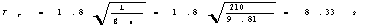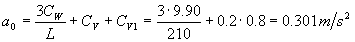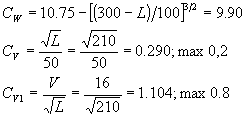The combined sway/yaw acceleration is given by: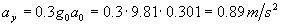The heave acceleration is given by: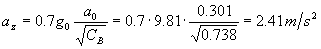Roll motion and acceleration: {B400}

Roll angle is given by: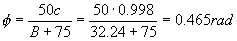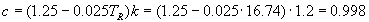The period of roll is given by: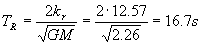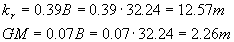z = distance from the baseline to the roll axis of rotation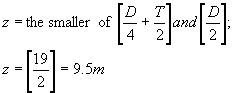The tangential roll acceleration at the height, h above the baseline is given by: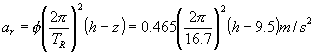Pitch motion and acceleration: {B500}

Pitch angle is given by: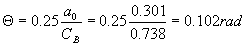The period of pitch is given by: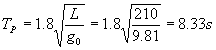The tangential pitch acceleration at the length, l from the A.P. is given by: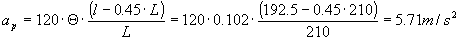Combined vertical acceleration: {B600}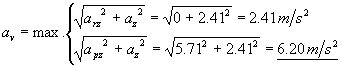Combined transverse acceleration: {B700}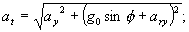Since we want to have ary given as an angular acceleration and ay is small compared to the other terms, the transverse acceleration is calculated as: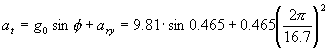ForsatCombined longitudinal acceleration: {B800}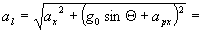Forsat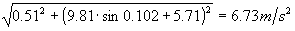### Combination between acceleration in different directions

Vertical and transverse force: {C500}

Vertical force: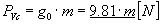Transverse force: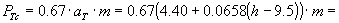Forsat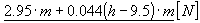Vertical and longitudinal force:

Vertical force: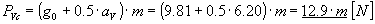Longitudinal force: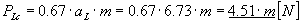[Front page] [Contents] [Previous] [Next] [Top]